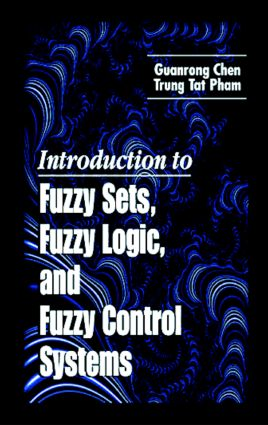# Introduction to Fuzzy Sets, Fuzzy Logic, and Fuzzy Control Systems

## 1st Edition

CRC Press

328 pages

##### Purchasing Options:\$ = USD
Hardback: 9780849316586
pub: 2000-11-27
\$185.00
x
eBook (VitalSource) : 9780429191442
pub: 2000-11-27
from \$28.98

FREE Standard Shipping!

### Description

In the early 1970s, fuzzy systems and fuzzy control theories added a new dimension to control systems engineering. From its beginnings as mostly heuristic and somewhat ad hoc, more recent and rigorous approaches to fuzzy control theory have helped make it an integral part of modern control theory and produced many exciting results. Yesterday's "art" of building a working fuzzy controller has turned into today's "science" of systematic design.

To keep pace with and further advance the rapidly developing field of applied control technologies, engineers, both present and future, need some systematic training in the analytic theory and rigorous design of fuzzy control systems. Introduction to Fuzzy Sets, Fuzzy Logic, and Fuzzy Control Systems provides that training by introducing a rigorous and complete fundamental theory of fuzzy sets and fuzzy logic, and then building a practical theory for automatic control of uncertain and ill-modeled systems encountered in many engineering applications. The authors proceed through basic fuzzy mathematics and fuzzy systems theory and conclude with an exploration of some industrial application examples.

Almost entirely self-contained, Introduction to Fuzzy Sets, Fuzzy Logic, and Fuzzy Control Systems establishes a strong foundation for designing and analyzing fuzzy control systems under uncertain and irregular conditions. Mastering its contents gives students a clear understanding of fuzzy control systems theory that prepares them for deeper and broader studies and for many practical challenges faced in modern industry.

### Reviews

"The book would certainly provide a sound and systematic introduction…Clearly and thoroughly written, it admirably meets the authors' stated goals and definitely deserves a place in the library of all institutions where courses on fuzzy systems and allied topics are taught. An excellent introduction to these fields via self-study."

-R. Bharath, CHOICE, September 2001

"Overall, the text is very well written and provides a rigorous analytical approach to fuzzy systems. The topics are laid out in a logical sequence where later chapters build on the ideas of the earlier ones. Solved examples at the end of the chapters do a good job of clarifying the concepts in the body of these chapters. This reviewer recommends… either as a textbook on fuzzy control or as a companion reference for a more general course on intelligent control. The book also belongs on the shelves of engineering libraries of both industry and academia."

-Applied Mechanics Review, vol. 54, no. 6, November 2001

FUZZY SET THEORY

Classical Set Theory

Fuzzy Set Theory

Interval Arithmetic

Operations on Fuzzy Sets

FUZZY LOGIC THEORY

Classical Logic Theory

The Boolean Algebra

Multi-Valued Logic

Fuzzy Logic and Approximate Reasoning

Fuzzy Relations

Fuzzy Logic Rule Base

FUZZY SYSTEM MODELING

Modeling of the Static Fuzzy Systems

Stability Analysis of Discrete-Time Dynamic Fuzzy Systems

Modeling of Continuous-Time Dynamic Fuzzy Systems

Stability Analysis of Continuous-Time Fuzzy Systems

Controllability Analysis of Continuous-Time Dynamic Fuzzy Systems

Analysis of Nonlinear Continuous-Time Dynamic Fuzzy Systems

FUZZY CONTROL SYSTEMS

Classical Programmable Logic Control

Fuzzy Logic Control I: A General Model-Free Approach

Fuzzy Logic Control II: A General Model-Based Approach

FUZZY PID CONTROLLERS

Conventional PID Controllers

Fuzzy PID Controllers

Fuzzy PID Controllers: Stability Analysis

Fundamental Adaptive Fuzzy Control Concept

Gain Scheduling

Fuzzy Self-Tuning Regulator

Model Reference Adaptive Fuzzy Systems

Dual Control

Sub-Optimal Fuzzy Control

APPLICATIONS IN FUZZY CONTROL

Health Monitoring Fuzzy Diagnostic Systems

Fuzzy Control of Image sharpness for Auto-focus Cameras

Fuzzy Control for Servo Mechanic Systems

Fuzzy PID Controllers for Servo Mechanic Systems

Fuzzy Controllers for Robotic Manipulator

Note: Each chapter also contains Problems and References

### Subject Categories

##### BISAC Subject Codes/Headings:
MAT003000
MATHEMATICS / Applied
SCI055000
SCIENCE / Physics
TEC007000
TECHNOLOGY & ENGINEERING / Electrical